DATA MINING
Desktop Survival Guide
by Graham Williams[#!freund.schapire:1995:decis_theor!#] introduced AdaBoost, popularising the idea of ensemble learning where a committee of models cooperate to deliver a better outcome. For a demonstration of AdaBoost visit http://www1.cs.columbia.edu/~freund/adaboost/.

The original formulation of the algorithm, as in [#!freund.schapire:1995:decis_theor!#], adjusts all weights each iteration. Weights are increased if the corresponding record is misclassified by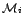or decreased if it is correctly classified by. The weights are then further normalised each iteration to ensure they continue to represent a distribution (so that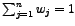). This can be simplified, as by hastie.tibshirani.etal:2001:stats_learn, to only increase the weights of the misclassified entities. We use this simpler formulation in the above description of the algorithm. Consequently, the calculation of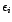(line 9) includes the sum of the weights as a denominator (which is 1 in the original formulation). Only the weights associated with the misclassified entities are modified in line 11. The original algorithm modified all weights by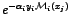which equates to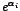for misclassified entities (since either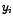or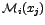is -1, but not both) and to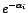for correctly classified entities (since bothorare either 1 or -1). For each iteration the new weights in the original algorithm are normalised by dividing each weight by a calculated factor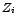.

Cost functions other than the exponential loss criterion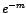have been proposed. These include the logistic log-likelihood criterion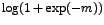used in LogitBoost),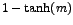(Doom II) and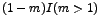(Support Vector Machines).